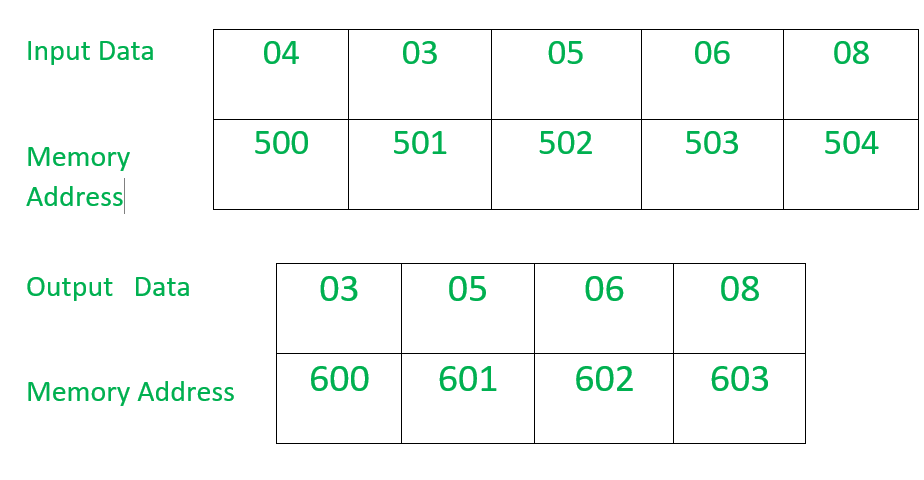# 8086 program to transfer a block of bytes by using string instruction

Problem – Write an assembly language program to transfer a block of bytes from one memory location to another memory location by using string instruction.

Example –Example –

1. In this example, the counter value stored in CX register is 4.
2. The block of data which is stored from memory location starting from 501 to 504 offset is transferred to another memory location which is starting from 600 to 603 offset.

Assumptions –

• The value of counter which tells the number of bytes to be transferred is stored at offset 500.
• The 8-bit data which have to be transfer is stored in continuous memory location starting from 501.
• The data is transferred to a continuous memory location starting from 600.
• The value of DS and ES is taken equal to 0000.
• the program starts from offset 400.
• CLD instruction is used to clear the directional flag, i.e., DF=0. Now, value of SI and DI will be increased.

``` SI=SI+1
DI=DI+1 ```

REP instruction is used to repeat the step until value of CX is not equal to zero and value of CX is decremented by one at every step, i.e.,

`CX=CX-1 `

MOVSB instruction is used to transfer bytes only from source memory location (MADS) to destination memory location (MAES).

```MADS-->MAES
MAES=ES*10+DI ```

Here, value of SI and DI is updated automatically.

`if DF=0, SI=SI+1 and DI=DI+1  `

Algorithm –

1. set the value of offset SI equal to 500.
2. set the value of offset DI equal to 600.
3. load the value 0000 into register AX.
4. load the data of AX register into DS(data segment).
5. load the data of AX register into ES(extra segment).
6. load the data of offset SI into CL register and load value 00 into CH register.
7. increment the value of SI by one.
8. clear the directional flag so that data is read from lower memory to higher memory location.
9. check the value of CX, if not equal to zero then repeat step 10 otherwise go to step 11.
10. transfer the data from source memory location to destination memory location and decrease the value of CX by one.
11. Stop.

Program –

0400 MOV SI, 500 SI<-500
0403 MOV DI, 00 DI<-600
0406 MOV AX, 0000 AX<-0000
0409 MOV DS, AX DS<-AX
040B MOV ES, AX ES<-AX
040D MOV CL, [SI] CL<-[SI]
0410 MOV CH, 00 CH<-00
0412 INC SI SI<-SI+1
0413 CLD clears the directional flag
0414 REP repeat until CX is not equal to zero
and CX=CX-1 at every step
0415 MOVSB transfer the data from source to destination memory location
0416 HLT end

Explaination –

1. MOV SI, 500: load the value 500 into offet SI.
2. MOV DI, 600: load the value 600 into offset DI.
3. MOV AX, 0000: load the value 0000 into AX register.
4. MOV DS, AX: load the value of AX register into DS (data segment).
5. MOV ES, AX: load the value of AX register into ES (extra segment).
6. MOV CL, [SI]: load the data of offset SI into CL register.
7. MOV CH, 00: load value 00 into CH register.
8. INC SI: increment the value of SI by one.
9. CLD: clears the directional flag i.e. DF=0.
10. REP: repeat until value of CX is not equal to zero and decrement the value of CX by one at each step.
11. MOVSB: transfer the data from source memory location to destination memory location.
12. HLT: end.

My Personal Notes arrow_drop_upCheck out this Author's contributed articles.

If you like GeeksforGeeks and would like to contribute, you can also write an article using contribute.geeksforgeeks.org or mail your article to contribute@geeksforgeeks.org. See your article appearing on the GeeksforGeeks main page and help other Geeks.

Please Improve this article if you find anything incorrect by clicking on the "Improve Article" button below.

Improved By : Akanksha_Rai